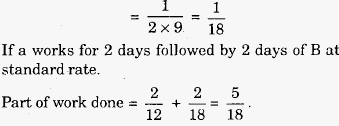# Engineering Maths - Time and Work

>>>>>>>>Time and Work

• A

4• B

12• C

14• D

16• Option : C
• Explanation :
A finishes the job at standard rate in 12 days.
So in 1 day, the part of job done by A = 1/12
Similarly the part of job done by B in 1 daySo, in (2 alternate days work of 'A' and 'B')
3 x 4 = 12 days
They will finish 3 x (5/18) = 15/18 th of the work.
Work remaining = 1 - (15/18) = 3/18 = 1/6
This is finished by A alone in= 2 days.
Hence, total number days = 12 + 2 = 14 days
Related Quiz.
Time and Work